﻿ Airfoil Parameterization Techniques: A Review

### Airfoil Parameterization Techniques: A Review

Nilesh P. Salunke, Juned Ahamad R. A., S.A. ChanniwalaOPEN ACCESSPEER-REVIEWED

## Airfoil Parameterization Techniques: A Review

Nilesh P. Salunke1, Juned Ahamad R. A.2,, S.A. Channiwala2

1Department of Mechanical Engineering, SVNIT, Surat, Gujarat, India

2Department of Mechanical Engineering, RCPIT, Shirpur, Maharashtra, India

### Abstract

Optimization of airfoil shape using evolutionary algorithms is becoming a trend in design of blades for turbomachines and aircraft. Evolutionary algorithms work with parameterization of airfoil shape, i.e. representation of airfoil with the help of some parameters which control its shape. Thus, one of the challenges in this field is to describe the airfoil with suitable parameters and explicit or implicit mathematical functions. This paper discusses various parameterization techniques being used to parameterize the airfoil.

### At a glance: Figures

123
Prev Next

• Salunke, Nilesh P., Juned Ahamad R. A., and S.A. Channiwala. "Airfoil Parameterization Techniques: A Review." American Journal of Mechanical Engineering 2.4 (2014): 99-102.
• Salunke, N. P. , A., J. A. R. , & Channiwala, S. (2014). Airfoil Parameterization Techniques: A Review. American Journal of Mechanical Engineering, 2(4), 99-102.
• Salunke, Nilesh P., Juned Ahamad R. A., and S.A. Channiwala. "Airfoil Parameterization Techniques: A Review." American Journal of Mechanical Engineering 2, no. 4 (2014): 99-102.

 Import into BibTeX Import into EndNote Import into RefMan Import into RefWorks

### 1. Introduction

Many parameterization techniques are in use nowadays. Some techniques prove to be advantageous over others with respect to convergence rate, range of airfoil that could be represented, etc. The further sections deal with various parameterization techniques explained briefly.

Any parameterization technique must satisfy the following three objectives.

•  It should minimize the number of degrees of freedom, i.e. number of parameters should be as less as possible.

•  It should be able to represent a wide range of existing airfoils.

•  Parameters should be simple to formulate and impose.

### 2. Bezier Parameterization

To represent an airfoil with the help of Bezier curve is one of the popular parameterization techniques. Airfoil consists of two curves namely camber line and thickness distribution. To obtain the upper and lower surfaces of the aerofoil, we add and subtract the thickness distribution to and from the camber line, respectively. A Bezier curve is controlled with the help of its control points in a plane. It passes through initial and final control points but it is not necessary for Bezier curve to pass through the each intermediate control point which is defining the shape of the aerofoil .

A n- degree Bezier curve is defined by (n+1) control points. A Three-degree Bezier curve will have four control points and will have shape as shown in Figure 1 depending on position of control points.

The blending functions of Bezier curves are Bernstein polynomials. It is denoted by Bin, where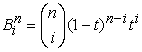(1)

where i=0, 1, 2, 3.

and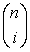is a binomial coefficient such that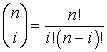(2)

For Bezier curve having n = 3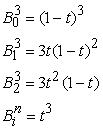(3)

Then, the Bezier curve associated with the given control points can be expressed as ,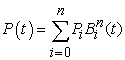(4)

### 3. Parsec Method

PARSEC is very common and highly effective method of airfoil parameterization. It uses eleven basic parameters to completely define the aerofoil shape as shown in Figure 2. The various parameters are leading edge radius (rLE), upper crest location (XUP,ZUP), lower crest location (XLO,ZLO), upper and lower curvature(ZxxUP,ZxxLO), trailing edge coordinate(ZTE) and directionTE),, trailing edge wedge angle(βTE) and thickness(∆ZTE).

In this method, a linear combination of shape functions describes the aerofoil shape.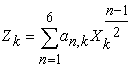(5)

With the help of defined geometric parameters, one can find coefficients an. The subscript k will take values 1 and 2 for upper and lower surface respectively. In this way, using these Parameters, one can control the maximum curvature on upper and lower surfaces and their location, which greatly affect the occurrence of shock wave and its strength. However, PARSEC does not provide sufficient control over the trailing edge shape where important flow phenomenon can take place because it fits a smooth curve between the maximum thickness point and the trailing edge which makes changes difficult at the trailing edge .

### 4. Sobieczky Method

As seen above, PARSEC method faces lack of control on trailing edge modeling, to overcome this, Sobieczky  proposed a way to give trailing edge a concave shape. Lower and upper surfaces observe the curvature increasing towards trailing edge. Such modified airfoil is known as divergent trailing edge .

As illustrated in Figure 3, in Sobieczky method, some portion of length of airfoil near the trailing edge undergoes increment in trailing edge thickness ∆Z. The parameters ∆α, L1, L2 govern the thickness ∆Z added to airfoil surface. Camber added to the upper and lower surfaces is controlled by the parameter ∆α.

Thickness added ∆Z is given by,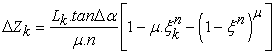(6)

where k is the subscript which takes values 1 and 2 to indicate upper and lower Surfaces, respectively. ξk is the x coordinate variable indicating chord length. Parameters n and μ can have various values. Lk is the length of airfoil which is modified on corresponding side. In this way, airfoil can be presented by the combination of both the methods. PARSEC method is used for much of the airfoil and Sobieczky method near the trailing edge.

Increment in curvature near the trailing edge can produce a flow near the trailing edge, which has a desirable pressure gradient on the airfoil surface . This pressure distribution increases the lift, due to decrease in upper surface camber. This method was investigated by authors in .

### 5. Modified Sobieczky Method

As seen above, the Sobieczky method modifies airfoil near trailing edge, but it cannot ensure a physically acceptable or feasible trailing edge. This method may result in overlapping of upper and lower surfaces as shown in Figure 4 by continuous line.

To overcome this drawback, modified Sobieczky method is introduced. In this method, upper surface is created first and then constraint on lower surface is imposed to finish up at trailing edge of the upper surface. Airfoil with dotted line in Figure 4 shows the modified airfoil shape which is physically acceptable .

### 6. New Parameterization Method

This method is proposed by the authors in Ref.  The problem associated with the modified Sobieczky method is that it tends to pull the trailing edge downward to increase the curvature near the trailing edge. It may induce the undesirable pressure gradient on the upper surface in viscous flow. It can be overcome by flattening the upper surface of the airfoil which creates weaker shock wave on the airfoil. Thus, for the shape of upper surface, new function for ∆Z is introduced given by following equation.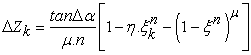(7)

Where, η is set to 0.8 and n is set to 6.

As can be seen in Eq.(7), parameter Lk of Eq.(6) is absent in this equation. It is due to fact that in this method not only a specified length near the trailing edge is modified but full upper surface is exposed to changes in Z coordinate. Now in this method ∆Z is computed from Eq.(7) for both upper and lower surfaces. This method is proved to be advantageous for modeling of upper surface but not for the lower surface. This method suffers the inability to make sufficient changes in the curvature of the lower surface compared to the modified Sobieczky method. It may reduce the lift. Hence to model lower surface, modified Sobieczky method is used and upper surface is modeled with the help of new parameterization method. Figure 5 illustrates how different methods described above affect the shape of airfoil .

### 7. Bezier-Parsec Parameterization

This method is a combination of Bezier and PARSEC parameterization techniques, which avails advantages of both the techniques. The Bezier-PARSEC parameterization uses PARSEC variables as parameters, which are used to define four separate Bezier curves. These four curves define the leading edge and trailing edge of the camber line and thickness distribution. The Bezier-PARSEC parameterization uses second order continuity to join the leading and trailing edges. Bezier- PARSEC parameterization is denoted by BP ijkl where i and j represent the order of leading and trailing edge of thickness curve and k and l represent the order of leading and trailing edge of camber curve .

Oyama et al.  showed that Bezier- PARSEC parameterization increased the robustness and convergence speed for aerodynamic optimization using genetic algorithms .

BP 3333 parameterization

As name suggests, all the four curves of the Bezier-PARSEC Parameterization, i.e. leading and trailing edges of thickness curve & leading and trailing edges of camber curve have polynomials of degree three.

Parametrically a third degree Bezier curve is given by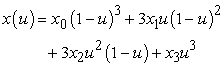and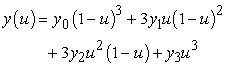(8)

Where, u varies from 0 to 1. The BP 3333 parameterization depends on the 12 aerodynamic parameters shown in Figure 6 – there are no free Bezier points in BP 3333.

The control points are given by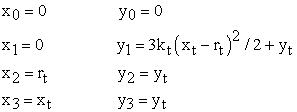Trailing edge thickness curve

The control points are given by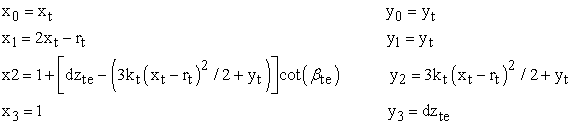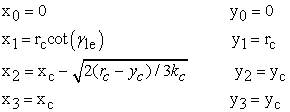The control points are given by

Trailing edge camber curve

The control points are given by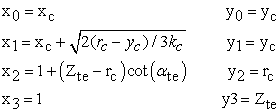BP 3434 parameterization

As name suggests, in BP 3434 parameterization both the leading edge curves have polynomials of degree three while both the trailing edge curves have the polynomials of degree four. The BP 3434 parameterization depends on ten aerodynamic parameters, plus five Bezier parameters. These are shown in Figure 7.

Figure 7. BP 3434 airfoil geometry and Bezier control points defined by ten aerodynamic and five Bezier parameters

Parametrically a fourth degree Bezier curve is given by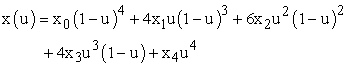and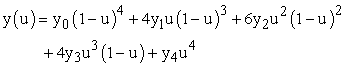(9)

The control points are given by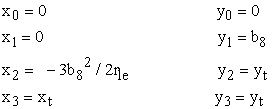Trailing edge thickness curve

The control points are given by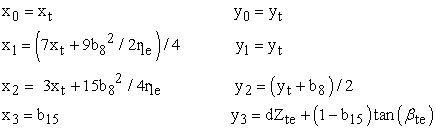The control points are given by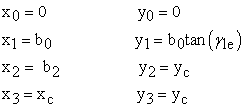Trailing edge camber curve

The control points are given by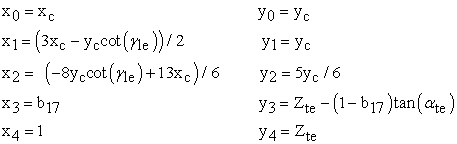.

### 8. Conclusion

Bezier parameterization is a Well-known parameterization technique. PARSEC method parameterizes the airfoil with maximum aerodynamic parameters. Combination of Bezier and PARSEC techniques has proved to be very advantageous and covering a wide range of airfoils. Combination of these two techniques combines the advantages of both the techniques. BP 3333 technique converges speedily due to less number of parameters involved. But BP 3333 does not control trailing edge very sharply due to less number of parameters. BP 3434 technique overcomes the drawback of BP 3333 but convergence speed reduces due to greater number of variable. High speed digital computers can compensate the slow convergence rate of BP 3434. Hence BP 3434 can be effectively applied for optimization of airfoil.

### References

  R.W. Derksen, Tim Rogalsky, “Bezier-PARSEC: An optimized aerofoil parameterization for design”, Advances in Engineering Software 41 (2010) 923-930.In article CrossRef  Ava Shahrokhi, Alireza Jahangirian, “Airfoil shape parameterization for optimum Navier–Stokes design with genetic algorithm”, Aerospace Science and Technology 11 (2007) 443-450.In article CrossRef  Prasanth. V, S. Anil Lal, “Bezier Parameterization of an airfoil using genetic algorithm”. http://hdl.handle.net/123456789/1026.In article  H. Sobieczky: Parametric Airfoils and Wings, in: Notes on Numerical Fluid Mechanics, pp.71-88, Vieweg (1998).In article  A. Shahrokhi, A.R. Jahangirian, N. Fouladi, Navier–Stokes optimization using genetic algorithm and a flexible parametric airfoil method, in: ERCOFTAC Conference on Design Optimization: Methods and Application, University of Las Palmas de Gran Canaria, Spain, April 2006.In article  Oyama A, Obayashi S, Nakahashi K. Fractional factorial design of genetic coding for aerodynamic optimization. AIAA Paper 99-3298; 1999.In article